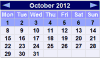# Probability: Complement

Complement of an Event: All outcomes that are NOT the event.When the event is Heads, the complement is TailsWhen the event is {Monday, Wednesday} the complement is {Tuesday, Thursday, Friday, Saturday, Sunday}When the event is {Hearts} the complement is {Spades, Clubs, Diamonds, Jokers}

So the Complement of an event is all the other outcomes (not the ones we want).

And together the Event and its Complement make all possible outcomes.

## Probability

Probability of an event happening = Number of ways it can happenTotal number of outcomes

### Example: the chances of rolling a "4" with a die

Number of ways it can happen: 1 (there is only 1 face with a "4" on it)

Total number of outcomes: 6 (there are 6 faces altogether)

So the probability = 16

The probability of an event is shown using "P":

P(A) means "Probability of Event A"

The complement is shown by a little mark after the letter such as A' (or sometimes Ac or A):

P(A') means "Probability of the complement of Event A"

The two probabilities always add to 1

P(A) + P(A') = 1

### Example: Rolling a "5" or "6"Event A is {5, 6}

Number of ways it can happen: 2

Total number of outcomes: 6

P(A) = 26 = 13

The Complement of Event A is {1, 2, 3, 4}

Number of ways it can happen: 4

Total number of outcomes: 6

P(A') = 4 6 = 2 3

P(A) + P(A')  =   1 3 + 2 3   =   3 3   =  1

Yep, that makes 1

It makes sense, right? Event A plus all outcomes that are not Event A make up all possible outcomes.

## Why is the Complement Useful?

It is sometimes easier to work out the complement first.### Example. Throw two dice. What is the probability the two scores are different?

Different scores are like getting a 2 and 3, or a 6 and 1. It is a long list:

A = { (1,2), (1,3), (1,4), (1,5), (1,6),
(2,1), (2,3), (2,4), (1,5), (1,6),
(3,1), (3,2), ... etc ! }

But the complement (which is when the two scores are the same) is only 6 outcomes:

A' = { (1,1), (2,2), (3,3), (4,4), (5,5), (6,6) }

And its probability is:

P(A') = 636 = 16

Knowing that P(A) and P(A') together make 1, we can calculate:

 P(A) = 1 − P(A') = 1 − 16 = 56

So in this case (and many others) it is easier to work out P(A') first, then calculate P(A) = 1 − P(A')